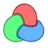# "Array of common values of the non-empty arrays"-question

Hello. I have a question:

I have 4 ui-spinners (which are blank Indeterminate: true) and 4 empty Arrays.
changing a spinner fills up an Array.

for example 3 spinner have a value:

```	Marr = #(1,2,3,4,5)
Rarr = #(3,4,5,6,7,8)  /*just changed */
Larr = #()
Tarr = #(4,5,6)```

I want to create a 5th Array which contains all the common values of the non-empty arrays

`    ComArr = #(4,5)  `

with 2 Array i can do:

```	ComArr = #()
If Marr.count >= 1 do (
Locarr = #()
for j=1 to Marr.count do (
N = findItem Rarr Marr[j]
if (N != 0) do (append Locarr Marr[j])
)
ComArr = Locarr
)else(
ComArr = Rarr
)```

But with multiple Array, you get a long list of findItem-expresions

Is there another way?

## Comment viewing options### .

You can use something like this(be sure to not include the empty bitarrays)

```(
Marr = #{1,2,3,4,5}
Rarr = #{3,4,5,6,7,8}
Larr = #{}
Tarr = #{4,5,6}

ComArr = Marr * Rarr * Tarr
)```### Sorry, it is not so long as i

Sorry, it is not so long as i thought:

```Marr = #(1,2,3,4,5)
Rarr = #(3,4,5,6,7,8)
Larr = #()
Tarr = #(4,5,6)
ComArr = #()

Free ComArr
If Marr.count >= 1 do (ComArr = Marr)

if Rarr.count >= 1 do (
Locarr = #()
for i=1 to Rarr.count do (
N = findItem ComArr Rarr[i]
if (N != 0) do (append Locarr Rarr[i])
)
ComArr = Locarr
)

if Larr.count >= 1 do (
Locarr = #()
for i=1 to Larr.count do (
N = findItem ComArr Larr[i]
if (N != 0) do (append Locarr Larr[i])
)
ComArr = Locarr
)

if Tarr.count >= 1 do (
Locarr = #()
for i=1 to Tarr.count do (
N = findItem ComArr Tarr[i]
if (N != 0) do (append Locarr Tarr[i])
)
ComArr = Locarr
)```

still, if someone knows a better way (appendIfNOTUnique) i would like to learn :)### if the data are positive

if the data are positive integer , miauu had told you the best method
if not , you must travel all elements , no way else
for more elegant code , you could use two-dimensional array and function to travel

```fn GetArrayAinB Asel Bsel =
(
if Asel.count == 0 or Bsel.count == 0 then
#()
else
for i in Asel where finditem Bsel i > 0 collect i
)
sel=#(#(),#(),#(),#())
comarr=#()
sel = #(1,2,3,4,5)
sel = #(3,4,5,6,7,8)
sel = #()
sel = #(4,5,6)
for i = 1 to sel.count where sel[i].count > 0 do
(
comarr = sel[i]
for j = i + 1 to sel.count where comarr.count > 0 and sel[j].count > 0 do
comarr = GetArrayAinB comarr sel[j]
exit
)
comarr```

Automatic Efficient Intelligent

We change the work state### if the data are positive

if the data are positive integer , miauu had told you the best method
if not , you must travel all elements , no way else
for more elegant code , you could use two-dimensional array and function to travel

```fn GetArrayAinB Asel Bsel =
(
if Asel.count == 0 or Bsel.count == 0 then
#()
else
for i in Asel where finditem Bsel i > 0 collect i
)
sel=#(#(),#(),#(),#())
comarr=#()
sel = #(1,2,3,4,5)
sel = #(3,4,5,6,7,8)
sel = #()
sel = #(4,5,6)
for i = 1 to sel.count where sel[i].count > 0 do
(
comarr = sel[i]
for j = i + 1 to sel.count where comarr.count > 0 and sel[j].count > 0 do
comarr = GetArrayAinB comarr sel[j]
exit
)
comarr```

Automatic Efficient Intelligent

We change the work state